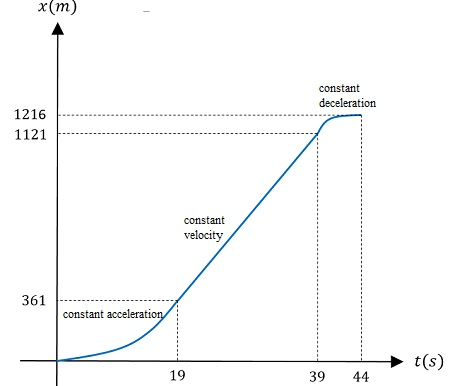# A truck on a straight road starts from rest, accelerating at 2.00 m/s^2 until it reaches a speed...

## Question:

A truck on a straight road starts from rest, accelerating at 2.00 m/s{eq}^2 {/eq} until it reaches a speed of 38.0 m/s. Then the truck travels for 20.0 s at a constant speed until the brakes are applied, stopping the truck in a uniform manner in an additional 5.00 s. Sketch a graph of the truck's displacement versus time.

a. Define the displacement coordinate in a sensible way.

b. Label axes clearly. Include symbols and units.

c. Label important events.

d. Use tick marks along the axes to indicate when and where the important events happened.

e. Indicate velocities and accelerations with suitable labels.

f. Does the displacement-time graph consist of straight lines, parabolas, or both?

g. Which takes longer for the truck - getting up to speed, or braking to a stop? Does your sketch show this?

## Graphs in kinematics

The solution of one-dimensional kinematic problems is analogous to the description of a real-valued function,

{eq}x=x(t) {/eq},

the law of motion for the body.

Physically, this function and its derivative must be continuous. Plotting {eq}x(t) {/eq} provides all the information required in the motion description. The slope in the position vs time graph is the velocity, since,

{eq}v(t)=\dfrac{dx}{dt} {/eq},

is the position's derivative respect to time.

The acceleration, on the other hand, is determined from the position's second derivative,

{eq}a(t)=\dfrac{d^2x}{dt^2} {/eq},

that is the concavity in the {eq}x {/eq} vs {eq}t {/eq} graph.

The graphical solution is then completely equivalent to the analytic one.

To draw the position vs time graph we need to compute the position of the truck at each notable time instant:

• When the truck reaches the velocity {eq}v=38 \;\rm m/s {/eq} after accelerating with {eq}a_1=2 \;\rm m/s^2 {/eq} from rest.

For this constant acceleration motion the velocity vs time equation yields,

{eq}v=a_1 \Delta t_1 {/eq},

since the initial velocity is zero.

Solving for the time interval,

{eq}\Delta t_1=\dfrac{v}{a_1}=\dfrac{38 \;\rm m/s}{2 \;\rm m/s^2}=19 \;\rm s {/eq}.

The displacement in this first interval is,

{eq}\Delta x_1=\dfrac{1}{2}a\Delta t_1^2=\dfrac{1}{2}\cdot 2 \;\rm m/s^2 \cdot 19^2 \;\rm s^2=361 \;\rm m {/eq}.

• In the second interval the truck moves at constant velocity {eq}v=38 \;\rm m/s {/eq} for {eq}\Delta t_2= 20 \;\rm s {/eq}.

The displacement is then,

{eq}\Delta x_2=v\Delta t_2=760 \;\rm m {/eq}.

The position after the second interval is the sum of the two displacements,

{eq}x_2=\Delta x_1+\Delta x_2=1121 \;\rm m {/eq}.

• In the third and last interval the truck decelerates from {eq}v=38 \;\rm m/s {/eq} to rest is {eq}\Delta t_3=5 \;\rm s {/eq}.

The acceleration in this motion can be computed as,

{eq}a_3=-\dfrac{v}{\Delta t_3}=-7.6 \;\rm m/s^2 {/eq}.

The displacement in this interval is,

{eq}\Delta x_3=v\Delta t_3-\dfrac{1}{2}a_3\Delta t_3^2 {/eq},

evaluating,

{eq}\Delta x_3=38 \;\rm m/s \cdot 5 \;\rm s-\dfrac{1}{2} \cdot 7.6 \;\rm m/s^2 \cdot 25 \;\rm s^2=95 \;\rm m {/eq}.

The total displacement in the motion is,

{eq}\Delta x_T=x_3=\Delta x_1+\Delta x_2+\Delta x_3=1216 \;\rm m {/eq}.

Using this information we can construct the graph.The first interval is a concave parabola for the accelerated motion, the second a straight line for the constant velocity motion and, finally, a convex parabola as the truck decelerates to rest at a constant rate.

The truck accelerates for {eq}\Delta t_1=19 \;\rm s {/eq} and decelerates for {eq}\Delta t_3=5 \;\rm s {/eq} (as can be seen from the graph).

It is very important to notice that the graph is continuous and so is its derivative (no sharp edges in the graph).